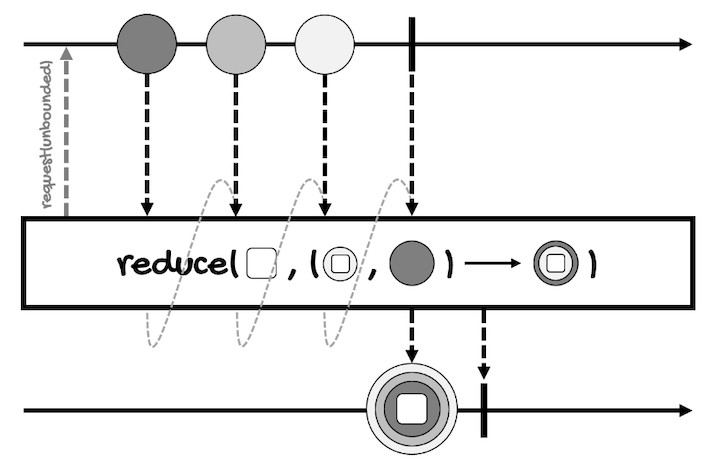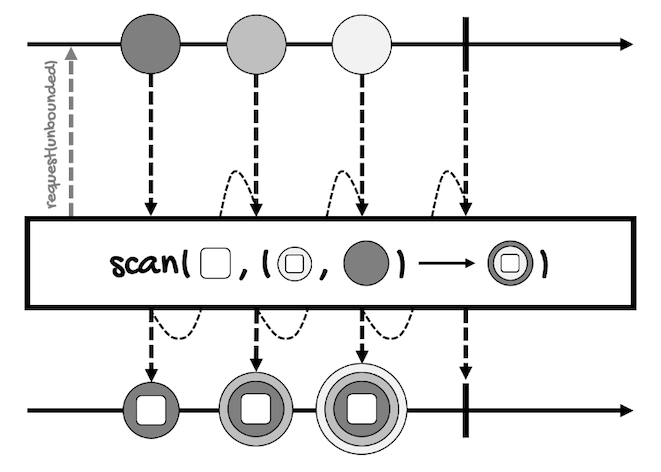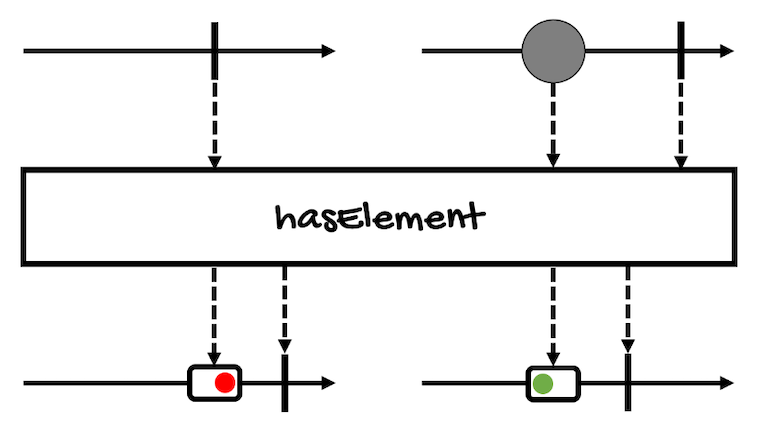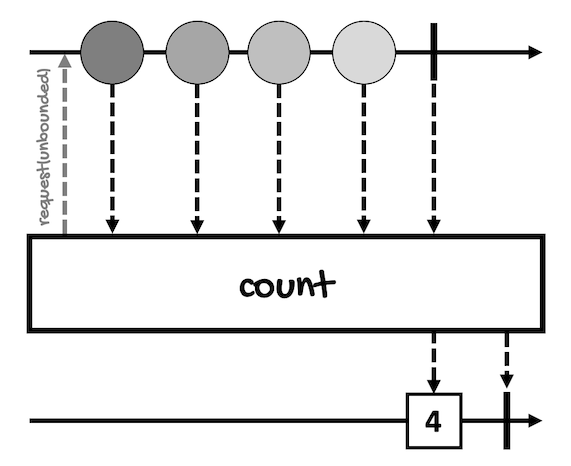# Aggregating a Flux

Do you remember the map-filter-reduce pattern/model?

Well, we have talked about the `map`, `filter`, and other related operators. Now it’s time to talk about `reduce`.

Reduce is an aggregation operation. An aggregation operation groups a collection of values to compute a single value.

In this lesson, we’ll review the following aggregation operators:

These operators are only available for `Flux`. Think about it, do they make sense for `Mono`?

## reduce

As the name implies, this operator reduces the values of a `Flux` into a single object.

There are three versions of `reduce`:

``````Mono<A> reduce(
A initial,
BiFunction<A,? super T,A> accumulator
)

Mono<T> reduce(
BiFunction<T,T,T> aggregator
)

Mono<A> reduceWith(
Supplier<A> initial,
BiFunction<A,? super T,A> accumulator
)
``````

The first version takes the initial value that will be passed to the `BiFunction`, the function in charge of computing the single value, along with the first value of the `Flux`. This will return an intermediary value that will be passed along to the `BiFunction` with the next value of the `Flux` and so on.

In any case, notice two things:

• The value returned by the `reduce` operator is wrapped in a `Mono`.
• The type of the initial value (for the versions that take an initial value) is represented by `A`. The returned `Mono` also has a type `A`, which means that the reduce function can convert the elements from type `T` to type `A`.

For instance, this is the marble diagram for the version that provides an initial value:Here’s an example where the reducing function adds all the elements of a `Flux` of integers:

``````Flux<Integer> integerFlux =
Flux.just(1, 2, 3);

integerFlux
.reduce(10, (a, b) -> {
int result = a + b;
System.out.format(
"[%d + %d] = %d\n", a, b, result
);
return result;
})
.subscribe(System.out::println);
}
``````

Of course, the reducing function could be as simple as `(a, b) -> a + b`, but in the example, I’ve added a statement to print the values of the arguments so you can see all the operations performed by the function.

Here’s the result:

``````[10 + 1] = 11
[11 + 2] = 13
[13 + 3] = 16
16
``````

Our initial value is `10`, so the first operation is `10 + 1`. The result, `11`, is carried over to the next iteration.

This way, the function receives `11` and `2`, returning `13` as the result.

Finally, the function receives `13` and `3`, which gives us the final result, `16`.

To try the second version of the `reduce` operator, we just need to remove the first argument:

``````Flux<Integer> integerFlux =
Flux.just(1, 2, 3);

integerFlux
.reduce((a, b) -> {
int result = a + b;
System.out.format(
"[%d + %d] = %d\n", a, b, result
);
return result;
})
.subscribe(System.out::println);
}
``````

Since we don’t have an initial value to work with, the first two elements of the `Flux` will be passed to the function.

Here’s the result:

``````[1 + 2] = 3
[3 + 3] = 6
6
``````

Finally, for the third version, `reduceWith`, we can wrap the initial value in a Supplier, which will be called on subscription and passed to the the `BiFunction`.

Here’s the first example modified to use `reduceWith`:

``````Flux<Integer> integerFlux =
Flux.just(1, 2, 3);

integerFlux
.reduceWith(() -> 10, (a, b) -> {
int result = a + b;
System.out.format(
"[%d + %d] = %d\n", a, b, result
);
return result;
})
.subscribe(System.out::println);
``````

The result will be the same:

``````[10 + 1] = 11
[11 + 2] = 13
[13 + 3] = 16
16
``````

## scan

This operator works the same as `reduce`. Actually, all versions of `scan` are identical to the versions of `reduce`:

``````Flux<A> scan(
A initial,
BiFunction<A,? super T,A> accumulator
)

Flux<T> scan(
BiFunction<T,T,T> accumulator
)

Flux<A> scanWith(
Supplier<A> initial,
BiFunction<A,? super T,A> accumulator
)
``````

The only difference is that `scan` emits each intermediary value.

For instance, this is the marble diagram for the version that provides an initial value:Here’s an example for the version that takes an initial value:

``````Flux<Integer> integerFlux =
Flux.just(1, 2, 3);

integerFlux
.scan(10, (a, b) -> a + b)
.subscribe(System.out::println);
``````

This is the result:

``````10
11
13
16
``````

Here’s the example for the second version:

``````Flux<Integer> integerFlux =
Flux.just(1, 2, 3);

integerFlux
.scan((a, b) -> a + b)
.subscribe(System.out::println);
``````

This is the result:

``````1
3
6
``````

And for `scanWith`:

``````Flux<Integer> integerFlux =
Flux.just(1, 2, 3);

integerFlux
.scanWith(() -> 10, (a, b) -> a + b)
.subscribe(System.out::println);
``````

This is the result:

``````10
11
13
16
``````

## collect

`collect*` operators allow you to collect all the elements of a `Flux` in a container, which can be a List, a Map, or another one implementing the Collector<T,A,R> interface.

Here are some examples of the available `collect*` operators.

This one collects all elements of the `Flux` into a container using the `Collector` Java 8 Stream API:

``````Mono<R> collect(
Collector<? super T,A,? extends R> collector
)
``````

This one collects all elements of the `Flux` into a `List`:

``````Mono<List<T>> collectList()
``````

The following collects all elements of the `Flux` into a `Map`. The key is extracted from each element by applying the `keyExtractor` function, the value is extracted by the `valueExtractor` function, and if several elements map to the same key, the associated value will be the most recent element:

``````Mono<Map<K,V>> collectMap(
Function<? super T,? extends K> keyExtractor,
Function<? super T,? extends V> valueExtractor
)
``````

The following collects all elements of the `Flux` into a `Map` of key-`List` pairs. The key is extracted from each element by applying the `keyExtractor` function, the value is extracted by the `valueExtractor` function, and every element mapping to the same key is stored in the `List` associated to that key:

``````Mono<Map<K,Collection<V>>> collectMultimap(
Function<? super T,? extends K> keyExtractor,
Function<? super T,? extends V> valueExtractor
)
``````

And this one collects all elements of the `Flux` until it completes, and then sort them using the provided `Comparator` into a `List`:

``````Mono<List<T>> collectSortedList(
@Nullable Comparator<? super T> comparator
)
``````

All these operators generate an empty container if the source `Flux` is empty.

For instance, this is the marble diagram for the version `collect(Collector<? super T,A,? extends R> collector)`:Let’s review a few examples, so I can show you how to use `collectMap`, `collectMultimap`, and `collectSortedList`.

For `collectMap`, the next example uses the version that takes a key and a value extractor:

``````Flux<Integer> integerFlux =
Flux.just(11, 22, 33, 34);

Mono<Map<Integer, Integer>> monoMap =
integerFlux.collectMap(
i -> i / 10,
i -> i % 10
);

monoMap.subscribe(System.out::println);
``````

As the key, `monoMap` uses the tens part of each element. As the value, `monoMap` uses the remainder when we divide the value by ten.

This is the result:

``````{1=1, 2=2, 3=4}
``````

Notice that the third element (`33`) was replaced by the last one (`34`). Remember, if many elements map to the same key, the associated value will be the most recently emitted element.

If we don’t want this behavior, we have to use `collectMultimap`:

``````Flux<Integer> integerFlux =
Flux.just(11, 22, 33, 34);

Mono<Map<Integer, Collection<Integer>>> monoMap =
integerFlux.collectMultimap(
i -> i / 10,
i -> i % 10
);

monoMap.subscribe(System.out::println);
``````

In this case, the map contains a collection to preserve all the elements that map to the same key:

``````{1=, 2=, 3=[3, 4]}
``````

We can use `collectSortedList` to sort the elements of the `Flux`.

The following example sorts the elements in reverse order:

``````Flux<Integer> integerFlux =
Flux.just(1, 2, 3);

Mono<List<Integer>> monoSortedList =
integerFlux.collectSortedList(
Comparator.reverseOrder()
);

monoSortedList.subscribe(System.out::println);
``````

This is the result:

``````[3, 2, 1]
``````

Finally, notice that all these operators return the container inside of a `Mono`, meaning that, even though `List` or `Map` are not asynchronous types, the collection process is done asynchronously.

## hasElement(s)/all/any

These operators can be considered aggregation operations because they reduce the value of a sequence to a `boolean` value.

Here are the definitions of these operators.

For `Mono`, `hasElement` emits a single `boolean` value that indicates if the `Mono` has an element:

``````Mono<Boolean> hasElement()
``````

It’s the only operator in this lesson that is available for `Mono`.

For `Flux`, we have a `hasElement` operator that emits a single `boolean` value that indicates if any of the elements of the `Flux` is equal to the provided value:

``````Mono<Boolean> hasElement(T value)
``````

And a `hasElements` operator that emits a single `boolean` value that indicates if the `Flux` has, at least, one element:

``````Mono<Boolean> hasElements()
``````

As well as an `all` operator that emits a single `boolean` value if all values of the `Flux` match the predicate:

``````Mono<Boolean> all(Predicate<? super T> predicate)
``````

And an `any` operator that emits a single `boolean` value if any of the values of the `Flux` match the predicate:

``````Mono<Boolean> any(Predicate<? super T> predicate)
``````

Notice that all these operators return the `boolean` value wrapped in a `Mono`.

This is the marble diagram of this operator (for `Mono`):Here’s an example that prints `false`:

``````Mono<Integer> integerMono =
Mono.just(1);

integerMono
.filter(i -> i > 1)
.hasElement()
.subscribe(System.out::println);
``````

The `Flux` equivalent takes the value to test if any of the elements is equal to it:

``````Flux<Integer> integerFlux =
Flux.just(1, 2, 3);

integerFlux
.filter(i -> i > 2)
.hasElement(3)
.subscribe(System.out::println);
``````

The above example only filters out the first two elements so `hasElement(3)` returns `true`.

On the other hand, `hasElements` (only available for `Flux`), returns `true` if the `Flux` has, at least, one element, like in the following example:

``````Flux<Integer> integerFlux =
Flux.just(1, 2, 3);

integerFlux
.filter(i -> i > 2)
.hasElements()
.subscribe(System.out::println);
``````

Now, if you want to test, for example, if all the elements of a `Flux` are even, you can use the `all` operator:

``````Flux<Integer> integerFlux =
Flux.just(1, 2, 3);

integerFlux
.filter(i -> i > 2)
.hasElements()
.subscribe(System.out::println);
``````

In this case, the result is `false`.

And if you want to test, for example, if at least one value is even, use the `any` operator:

``````Flux<Integer> integerFlux =
Flux.just(1, 2, 3);

integerFlux
.any(i -> i % 2 == 0)
.subscribe(System.out::println);
``````

The result will be `true`.

## count

Finally, one the easiest operators for `Flux` you’ll ever find:

``````Mono<Long> count()
``````

This operator counts the number of values in a `Flux`.

This is the marble diagram for this operator:Here’s an example:

``````Flux<Integer> integerFlux =
Flux.just(1, 2, 3, 4, 5);

Mono<Long> integerMono = integerFlux.count();
integerMono.subscribe(System.out::println);
``````

The value is wrapped in a `Mono<Long>`, however, the output shouldn’t be a surprise:

``````5
``````